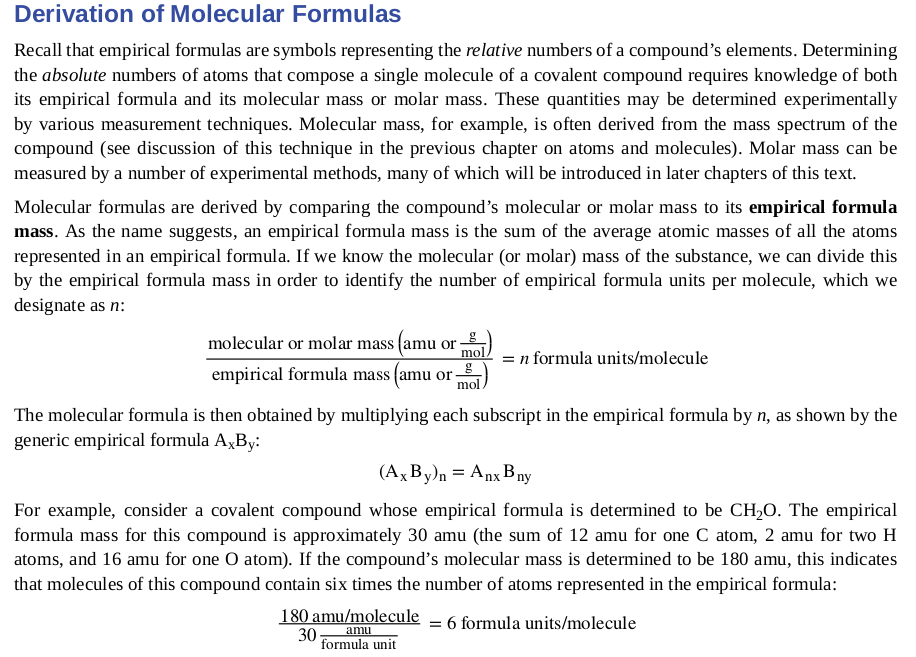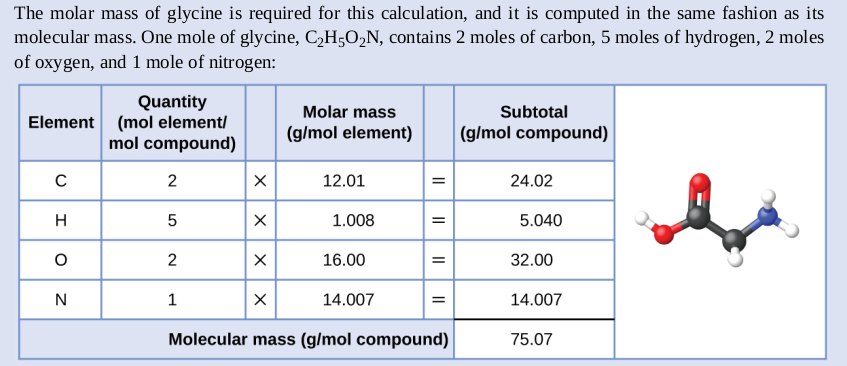April 16, 2021, 09:38:48 PM
Forum Rules: Read This Before Posting

###Topic: Derivation of molecular formulas - from where such number?  (Read 611 times)

0 Members and 1 Guest are viewing this topic.

####Jenran

• New Member
•• Posts: 3
• Mole Snacks: +0/-0##### Derivation of molecular formulas - from where such number?
« on: October 06, 2020, 08:12:11 AM »

I'm currently studying chemistry from textbook Chemistry (from OpenStax) and I'm stuck on this, trying to make sense out of it:Molecular formula is obtained by molar mass of element multiplied by quantity of that element and at end, adding together all elements like this:Empirical mass formula from what I read in this part of the book, is obtained basically in the same way, meaning that molecular mass for CH2O is 30 amu and empirical mass formula is also 30 amu, but in this book it is written that molecular mass is 180, I don't know what's going on, from where such a number? How is it even possible?

####Borek##### Re: Derivation of molecular formulas - from where such number?
« Reply #1 on: October 06, 2020, 08:46:55 AM »
You are misreading the book and/or misunderstanding/mistaking molecular formula with empirical formula.

Empirical formula tells you what is ratio of numbers of atoms in a molecule, it doesn't say anything about molecular mass and molecular formula (which can be any integer multiply of the experimental formula).
ChemBuddy chemical calculators - stoichiometry, pH, concentration, buffer preparation, titrations.info, pH-meter.info

####Jenran

• New Member
•• Posts: 3
• Mole Snacks: +0/-0##### Re: Derivation of molecular formulas - from where such number?
« Reply #2 on: October 12, 2020, 05:34:39 PM »
Empirical formula tells you what is ratio of numbers of atoms in a molecule, it doesn't say anything about molecular mass and molecular formula (which can be any integer multiply of the experimental formula).

I don't know if I am correct, but I think I realized that empirical formula mass is made up from empirical formula which is only a ratio of numbers of atoms, which means that "real" molecule is not CH2O but in this case, if molecular mass equals 180, it's actually C6H12O6.

But is CH2O and C6H12O6 same molecule? It's confusing.

####Borek##### Re: Derivation of molecular formulas - from where such number?
« Reply #3 on: October 13, 2020, 03:18:46 AM »
I don't know if I am correct, but I think I realized that empirical formula mass is made up from empirical formula which is only a ratio of numbers of atoms, which means that "real" molecule is not CH2O but in this case, if molecular mass equals 180, it's actually C6H12O6.

Sounds OK to me.

Quote
But is CH2O and C6H12O6 same molecule? It's confusing.

No, it is not the same molecule. But CH2O is the empirical formula of C6H12O6.

To make things less confusing consider that: CH2O is an empirical formula, but real formula of a real compound is (CH2O)n. It may happen that n=1, in which case CH2O is a real formula of a compound.

This is not invented to make life harder, this is just reflecting the realityTake two real and quite common compounds, one with a real, molecular formula CH2O (formaldehyde AKA methanal) and the second with a real, molecular formula C2H4O2 (acetic acid). If you make the elemental analysis you will find what are ratios of C:H:O, and you will find out both compounds have the same empirical formula of (CH2O)n. If you determine molar masses separately you will find out it is 30 g/mol for the first compound and 60 g/mol for the latter, so in the first case n=1 and in the second n=2. You can't determine n without knowing the molar mass.
« Last Edit: October 15, 2020, 03:22:50 AM by Borek »
ChemBuddy chemical calculators - stoichiometry, pH, concentration, buffer preparation, titrations.info, pH-meter.info

####Jenran

• New Member
•• Posts: 3
• Mole Snacks: +0/-0##### Re: Derivation of molecular formulas - from where such number?
« Reply #4 on: October 14, 2020, 05:43:18 PM »
I understand, thank you!